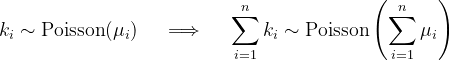﻿PoissonDistribution Class# PoissonDistribution Class

Represented a Poisson distribution.Inheritance Hierarchy

Namespace:  Meta.Numerics.Statistics.Distributions
Assembly:  Meta.Numerics (in Meta.Numerics.dll) Version: 4.1.4Syntax
`public sealed class PoissonDistribution : DiscreteDistribution`

The PoissonDistribution type exposes the following members.Constructors
NameDescriptionPoissonDistribution
Creates a new instance of a Poisson distribution.
TopProperties
NameDescriptionExcessKurtosis
Gets the excess kurtosis of the distribution.
(Overrides UnivariateDistributionExcessKurtosis.)Mean
Gets the mean of the distribution.
(Overrides DiscreteDistributionMean.)Skewness
Gets the skewness of the distribution.
(Overrides UnivariateDistributionSkewness.)StandardDeviation
Gets the standard deviation of the distribution.
(Inherited from UnivariateDistribution.)Support
Gets the interval over which the distribution is non-vanishing.
(Overrides DiscreteDistributionSupport.)Variance
Gets the variance of the distribution.
(Overrides UnivariateDistributionVariance.)
TopMethods
NameDescriptionCentralMoment
Gets a central moment of the distribution.
(Overrides DiscreteDistributionCentralMoment(Int32).)Cumulant
Computes a cumulant of the distribution.
(Overrides UnivariateDistributionCumulant(Int32).)Equals
Determines whether the specified object is equal to the current object.
(Inherited from Object.)ExpectationValue
Computes the expectation value of an artibrary function.
(Inherited from DiscreteDistribution.)GetHashCode
Serves as the default hash function.
(Inherited from Object.)GetRandomValue
Produces a random integer drawn from the distribution.
(Overrides DiscreteDistributionGetRandomValue(Random).)GetType
Gets the Type of the current instance.
(Inherited from Object.)InverseLeftProbability
Computes the value corresponding to the given percentile.
(Overrides DiscreteDistributionInverseLeftProbability(Double).)LeftExclusiveProbability
Computes the probability of obtaining a value less than the given value.
(Overrides DiscreteDistributionLeftExclusiveProbability(Int32).)LeftInclusiveProbability
Computes the probability of obtaining a value less than or equal to the given value.
(Overrides DiscreteDistributionLeftInclusiveProbability(Int32).)ProbabilityMass
Returns the probability of the obtaining the given value.
(Overrides DiscreteDistributionProbabilityMass(Int32).)RawMoment
Gets a raw moment of the distribution.
(Overrides DiscreteDistributionRawMoment(Int32).)RightExclusiveProbability
Computes the probability of obtaining a value greater than the given value.
(Overrides DiscreteDistributionRightExclusiveProbability(Int32).)ToString
Returns a string that represents the current object.
(Inherited from Object.)
TopRemarks

If events occur independely at uniformly distributed random times, the Poisson distribution gives the probabability distribution of the number of events than occur in a given time interval. If on average μ events occur in the time interval the number of actual events that occur is Poisson distributed with parameter μ.

The Poisson distribution has the unusual property that the distribution of a sum of Poisson distributed values itself has a poisson distribution, with a mean equal to the sum of the means of the contributing addends.See Also